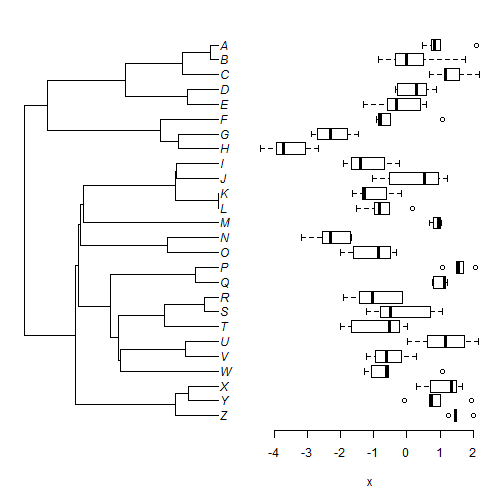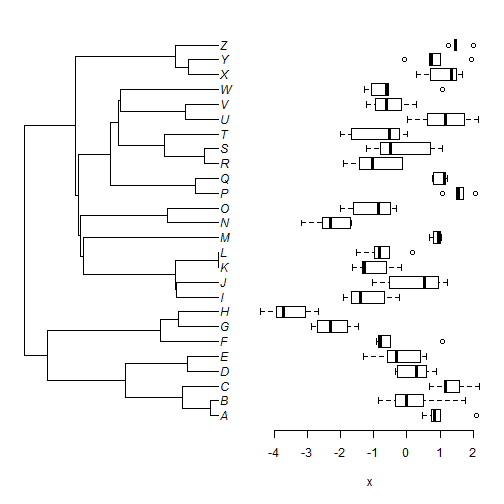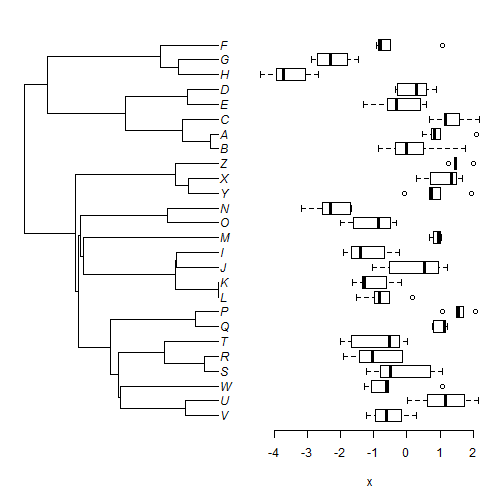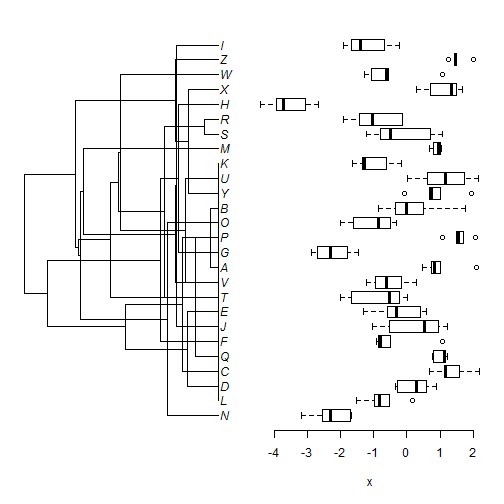## Monday, July 25, 2016

### Update to `plotTree.boxplot` for `formula` method

In the documentation to the new function `plotTree.boxplot` I noted:

“\code{x} can also be supplied as a formula, though in that case the factor levels need to be provided in the cladewise order of the tips in \code{tree}.”

What I didn't note is that if this is not done, then the result will be incorrect without any error being reported. This is because the `formula` provided by the user is passed directly to the function `boxplot` without any feedback to the tree plotter.

What I realized is that one thing that I could do was first run `boxplot.formula` with `plot=FALSE` which (invisibly) returns a list containing the order of the plotted boxes. Then I could pass that argument to `plotTree` using `tips`. Finally, I could re-run `boxplot` with `plot=TRUE`. If the order of the factor in `formula` is a valid cladewise order of the tips of the tree then the tree & boxplot will plot fine. If it is not, say if we randomize the levels of our factor, then the edges of the tree will cross when plotting.

Here is what I mean.

Here's our tree & data applied using the basic method:

``````library(phytools)
tree
``````
``````##
## Phylogenetic tree with 26 tips and 25 internal nodes.
##
## Tip labels:
##  Z, Y, X, W, V, U, ...
##
## Rooted; includes branch lengths.
``````
``````x[1:30] ## first 30 elements of x
``````
``````##            Z            Z            Z            Z            Z
##  1.481718482  1.999288805  1.244922289  1.465959575  1.439767680
##            Y            Y            Y            Y            Y
##  1.014757614  1.937999703  0.728102930  0.690196494 -0.081170095
##            X            X            X            X            X
##  1.506780336  1.680023687  1.337899661  0.300120161  0.706118301
##            W            W            W            W            W
## -0.615363928  1.062724934 -0.556182889 -1.070714742 -1.271925923
##            V            V            V            V            V
## -1.228228110 -0.957836899 -0.617736401  0.300541684 -0.161967102
##            U            U            U            U            U
##  1.722133482  1.169447096  0.624685958  2.154831186  0.009577268
``````
``````plotTree.boxplot(tree,x) ## basic method
``````Now let's try the formula method in which our factor is in the order of our tree's tip labels:

``````spp<-factor(names(x),levels=reorder(tree)\$tip.label)
plotTree.boxplot(tree,x~spp)
``````Next, we can flip the order of our factor levels. This is still a valid cladewise order so this should work fine:

``````spp<-factor(names(x),levels=tree\$tip.label[Ntip(tree):1])
spp
``````
``````##    Z Z Z Z Z Y Y Y Y Y X X X X X W W W W W V V V V V U U U U U T T T T T
##   S S S S S R R R R R Q Q Q Q Q P P P P P O O O O O N N N N N M M M M M
##   L L L L L K K K K K J J J J J I I I I I H H H H H G G G G G F F F F F
##  E E E E E D D D D D C C C C C B B B B B A A A A A
## Levels: A B C D E F G H I J K L M N O P Q R S T U V W X Y Z
``````
``````plotTree.boxplot(tree,x~spp)
````````````spp<-factor(names(x),untangle(ladderize(tree),"read.tree")\$tip.label)
spp
``````
``````##    Z Z Z Z Z Y Y Y Y Y X X X X X W W W W W V V V V V U U U U U T T T T T
##   S S S S S R R R R R Q Q Q Q Q P P P P P O O O O O N N N N N M M M M M
##   L L L L L K K K K K J J J J J I I I I I H H H H H G G G G G F F F F F
##  E E E E E D D D D D C C C C C B B B B B A A A A A
## Levels: V U W S R T Q P L K J I M O N Y X Z B A C E D H G F
``````
``````plotTree.boxplot(tree,x~spp)
``````(Note that we didn't ladderize our input tree for `plotTree.boxplot` - however the plotted tree will be ladderized.)

Finally, randomize them. This will not (generally speaking) result in a valid cladewise order:

``````spp<-factor(names(x),levels=sample(tree\$tip.label))
spp
``````
``````##    Z Z Z Z Z Y Y Y Y Y X X X X X W W W W W V V V V V U U U U U T T T T T
##   S S S S S R R R R R Q Q Q Q Q P P P P P O O O O O N N N N N M M M M M
##   L L L L L K K K K K J J J J J I I I I I H H H H H G G G G G F F F F F
##  E E E E E D D D D D C C C C C B B B B B A A A A A
## Levels: N L D C Q F J E T V A G P O B Y U K M S R H X W Z I
``````
``````plotTree.boxplot(tree,x~spp)
``````This makes it much easier to see if we have a problem using the `formula` method.

The update can be seen here.

1.2.